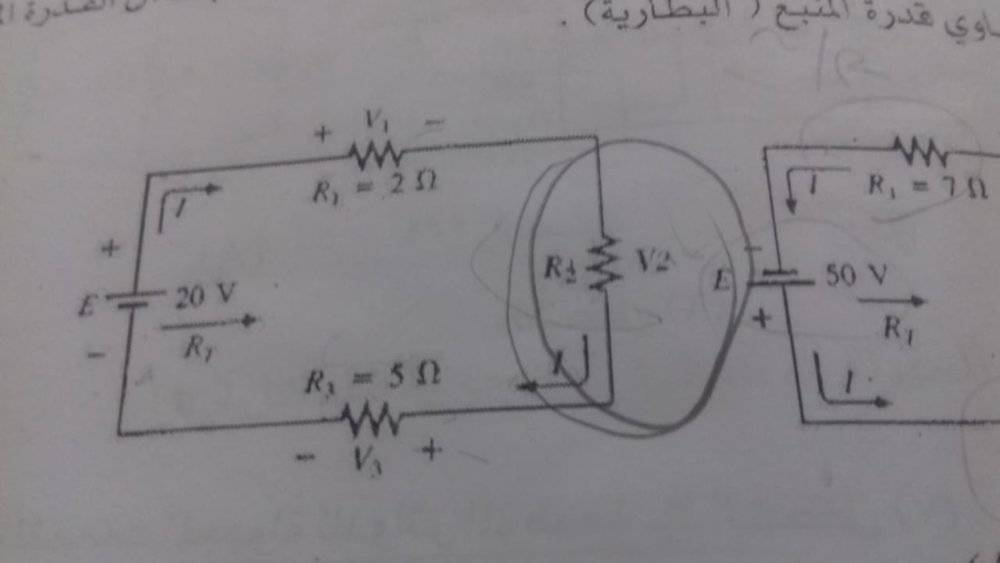# Find the unknown resistance in this series circuit

• Engineering
ahmed elshimy
Homework Statement:
Find r2 , v1 , v2 , v3 , It
Relevant Equations:
It = i1 = i2 = i3
V1 + v2 + v3 = E
How i can find r2 value in this circuit?
R1 = 2
R3 = 5
E = 20vGold Member
It looks like you already know that the same current is flowing through all of those resistors since they are in a series; you also have your equation above. Great job! $$I = i_1 = i_2 = i_3$$.

You've gotten to the next step using KVL. Great job there too! $$v_1 + v_2 + v_3 = E$$.

Just like a regular mathematics problem where you want to isolate the variable of interest... I would solve for v2 and apply an equation that relates resistance (R2) to voltage (v2) and current (I).

•ahmed elshimy
ahmed elshimy
It looks like you already know that the same current is flowing through all of those resistors since they are in a series; you also have your equation above. Great job! $$I = i_1 = i_2 = i_3$$.

You've gotten to the next step using KVL. Great job there too! $$v_1 + v_2 + v_3 = E$$.

Just like a regular mathematics problem where you want to isolate the variable of interest... I would solve for v2 and apply an equation that relates resistance (R2) to voltage (v2) and current (I).
Is there a way to actually solve it?
Or can't solve because didn't have any i or p ?

Mentor
Is there a way to actually solve it?
Or can't solve because didn't have any i or p ?
Are you given the value of I? You would need either I or the value of R2 to solve for the voltages and currents, it would seem. Did you copy the question exactly?

•ahmed elshimy
ahmed elshimy
Are you given the value of I? You would need either I or the value of R2 to solve for the voltages and currents, it would seem. Did you copy the question exactly?

Value of i not given...
Given r1, r3 , E.
Need r2 .
Yes i am copy exactly

Gold Member
You haven't provided enough information to solve for R2 yet.
You can see this by trying a couple of different values for R2, let's say R2=1 or R2=2. Then you can easily solve for all of the other circuit parameters. Then examine your solutions for some contradiction in the problem statement. This may help show you what you've left out in the given parameters, or, it may show you that the problem doesn't have a single solution.

•Joshy and berkeman
Gold Member
With the given information the only 'solution' would have to be symbolic; that is write the equation(s) for I2 in terms of R1, R3, E, R2. Since R1, R3, E are known, the equation will be:

{some number based on R1, R3, E} and some operator on R2.

You still have two unknowns R2 and I2. This shows that there is a 'universe' of solutions, that is you can pick any numeric value for either R2 or I2 and get a valid value for the other one... and then find the rest of the values.

To get the symbolic solutions for the other unknowns, the other requested equations will have I2, R2, or both of them in the solution taking the place of a number.

Well, a little long winded but I hope it helps.

Cheers,
Tom

•berkeman
Gold Member
I agree with Dave (response #6).

I and R2 maybe unknown, but wouldn't it be nice if there were some formula that could help you get the voltage across each resistor so that you could use your second equation :)?

Can the sum of the voltages across each resistor be more or less than E?